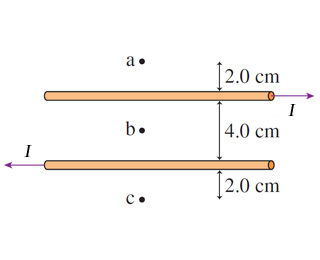# Problem: Assume that I = 11 A .Part AWhat is the magnetic field strength at point c?Part BWhat is the direction of the magnetic field strength at point c?

###### FREE Expert Solution

Magnetic field around a conductor:

$\overline{){\mathbf{B}}{\mathbf{=}}\frac{{\mathbf{\mu }}_{\mathbf{0}}\mathbf{i}}{\mathbf{2}\mathbf{\pi }\mathbf{r}}}$

Right-hand grip rule: The thumb points in the direction of the current, fingers curl in the direction of the magnetic field.

(a)

Using the right-hand rule, the magnetic field at c due to the top wire is into the page. The magnetic field due to the lower wire is out of the page.

100% (354 ratings)###### Problem Details

Assume that I = 11 A .Part A

What is the magnetic field strength at point c?

Part B

What is the direction of the magnetic field strength at point c?

Frequently Asked Questions

What scientific concept do you need to know in order to solve this problem?

Our tutors have indicated that to solve this problem you will need to apply the Magnetic Field Produced by Straight Currents concept. You can view video lessons to learn Magnetic Field Produced by Straight Currents. Or if you need more Magnetic Field Produced by Straight Currents practice, you can also practice Magnetic Field Produced by Straight Currents practice problems.

What professor is this problem relevant for?

Based on our data, we think this problem is relevant for Professor Shpyrko's class at UCSD.